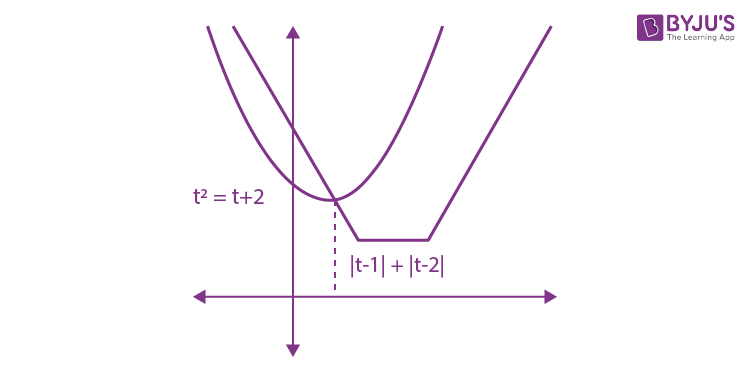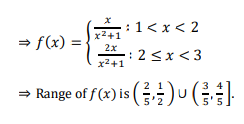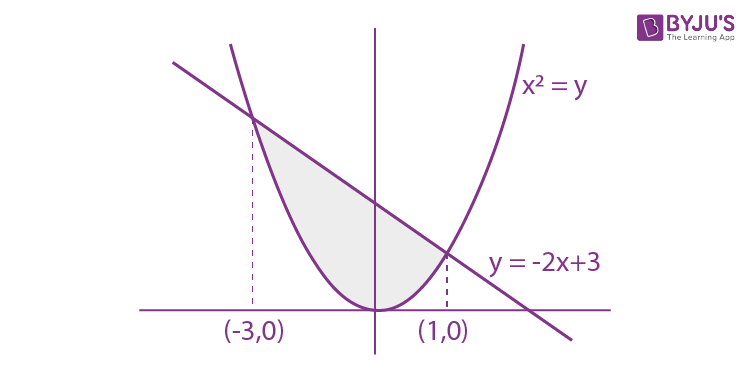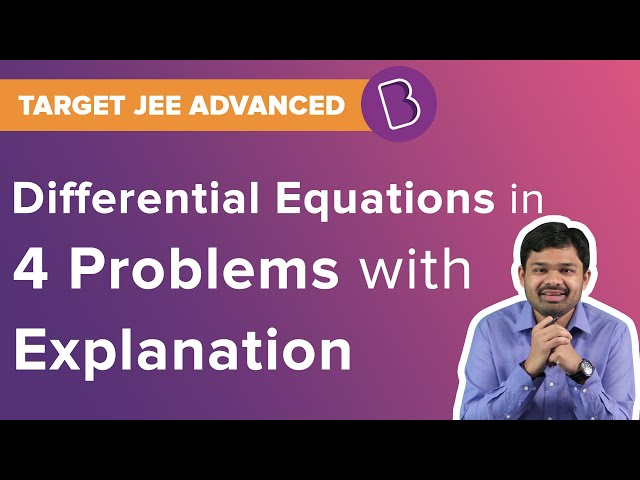Win up to 100% scholarship on Aakash BYJU'S JEE/NEET courses with ABNAT Win up to 100% scholarship on Aakash BYJU'S JEE/NEET courses with ABNAT

# JEE Main 2020 Paper with Solutions Mathematics 8th Jan Shift 2

JEE is an examination conducted every year for admission into various institutions in the country. This page includes the answers for all the questions asked in JEE Main 2020 Mathematics Solutions (Shift 2, 8th January). JEE Main has a fixed structure and is not subjected to change every year. Therefore, referring and practising these questions will help in scoring well in the examination.
January 8 Shift 2 – Maths
1. Let 𝐴 and 𝐵 be two events such that the probability that exactly one of them occurs is 2/5 and the probability that 𝐴 or 𝐵 occurs is 1/2, then the probability of both of them occur together is
a. 0.10
b. 0.20
c. 0.01
d. 0.02

P(𝐴 or 𝐵) = 𝑃(𝐴 ∪ 𝐵) = 1/2

P(exactly one of 𝐴 or 𝐵)= 2/5

⇒ P(𝐴) − 𝑃(𝐴 ∩ 𝐵) + 𝑃(𝐵) − 𝑃(𝐴 ∩ 𝐵) = 2/5

⇒ P(𝐴 ∪ 𝐵) − 𝑃(𝐴 ∩ 𝐵) = 2/5

⇒ P(𝐴 ∩ 𝐵) = 1/10

2. Let 𝑆 be the set of all real roots of the equation, 3x (3x − 1) + 2 = |3x − 1| + |3x − 2|. Then S:
a. is a singleton.
b. is an empty set.
c. contains at least four elements
d. contains exactly two elements.

3𝑥 (3𝑥 − 1) + 2 = |3 𝑥 − 1| + |3𝑥 − 2|

Let 3𝑥 = 𝑡

t(𝑡 − 1) + 2 = |𝑡 − 1| + |𝑡 − 2|

⇒ 𝑡 2 − 𝑡 + 2 = |𝑡 − 1| + |𝑡 − 2|

We plot 𝑡 2 − 𝑡 + 2 and |𝑡 − 1| + |𝑡 − 2|

As 3 𝑥 is always positive, therefore only positive values of 𝑡 will be the solution.Therefore, we have only one solution.

3. The mean and variance of 20 observations are found to be 10 and 4, respectively. On rechecking, it was found that an observation 9 was incorrect and the correct observation was 11. Then the correct variance is:
a. 4.01
b. 3.99
c. 3.98
d. 4.02

Mean = 10 ⇒ ∑ 𝑥𝑖/20 = 10 ⇒ ∑ 𝑥𝑖 = 200

Variance = 4 ⇒ ∑ 𝑥𝑖 2/20 − 100 = 4 ⇒ ∑ 𝑥𝑖 2 = 2080

New mean = (200−9+11) / 20 = 202/20 = 10.1

New variance = (2080−81+121)/ 20 − (10.1) 2

= 106 − 102.01 = 3.99

4. Let 𝑎⃗ = 𝑖̂− 2𝑗̂+ 𝑘̂, 𝑏⃗ = 𝑖̂− 𝑗̂+ 𝑘̂ be two vectors. If 𝑐⃗ is a vector such that 𝑏⃗× 𝑐⃗= 𝑏⃗× 𝑎⃗and 𝑐⃗. 𝑎⃗= 0 then 𝑐⃗. 𝑏⃗ is equal to:
a. 1/2
b. – 3/2
c. – 1/2
d. −1

𝑎⃗ = 𝑖̂ − 2𝑗̂ + 𝑘̂

𝑏⃗ = 𝑖̂ − 𝑗̂ + 𝑘̂

𝑏⃗× 𝑐⃗= 𝑏⃗× 𝑎⃗

⇒ 𝑎⃗ × ( 𝑏⃗ × 𝑐⃗ ) = 𝑎⃗× (𝑏⃗× 𝑎⃗)

⇒ (𝑎⃗. 𝑐⃗ ) 𝑏⃗ − (⃗𝑎⃗. 𝑏⃗)𝑐⃗= (𝑎⃗. 𝑎⃗)𝑏⃗ − (𝑎⃗. 𝑏⃗)𝑎⃗

⇒ − (⃗𝑎⃗. 𝑏⃗)𝑐⃗= (𝑎⃗. 𝑎⃗)⃗𝑏⃗ − (𝑎⃗. 𝑏⃗)𝑎⃗

⇒ −4𝑐⃗= 6(𝑖̂− 𝑗̂+ 𝑘̂) − 4(𝑖̂− 2𝑗̂+ 𝑘̂)

⇒ 𝑐⃗ = − 1/2 (𝑖̂ + 𝑗̂ + 𝑘̂)

∴ 𝑏⃗. 𝑐⃗= − ½

5. Let 𝑓: (1,3) → 𝑅 be a function defined by 𝑓(𝑥) = 𝑥[𝑥] /(𝑥 2+1) , where [𝑥] denotes the greatest integer ≤ 𝑥. Then the range of 𝑓 is:
a. ( 2/5 , 3/5 ] ∪ ( 3/4 , 4/5 )
b. ( 2/5 , 4/5 ]
c. ( 3/5 , 4/5 )
d. ( 2/5 , 1/2 ) ∪ ( 3/5 , 4/5 ]

f(𝑥) = 𝑥[𝑥]/(𝑥2+1)6. If 𝛼 and 𝛽 be the coefficients of 𝑥 4 and 𝑥 2 respectively in the expansion of
$$\begin{array}{l}(x+\sqrt{x^{2}-1})^{6}+(x-\sqrt{x^{2}-1})^{6}\end{array}$$
, then:

a. 𝛼 + 𝛽 = −30
b. 𝛼 − 𝛽 = −132
c. 𝛼 + 𝛽 = 60
d. 𝛼 − 𝛽 = 60

$$\begin{array}{l}(x+\sqrt{x^{2}-1})^{6}+(x-\sqrt{x^{2}-1})^{6}\end{array}$$

= 2[6𝐶0𝑥 6 + 6𝐶2𝑥 4 (𝑥2 − 1) + 6𝐶4𝑥 2(𝑥 2 − 1) 2 + 6𝐶6 (𝑥2 − 1)3]

= 2[32𝑥 6 − 48𝑥 4 + 18𝑥 2 − 1]

⇒ 𝛼 = −96, 𝛽 = 36

⇒ 𝛼 − 𝛽 = −132

7. If a hyperbola passes through the point (10, 16) and it has vertices at (±6, 0), then the equation of the normal at 𝑃 is:
a. 3𝑥 + 4𝑦 = 94
b. 𝑥 + 2𝑦 = 42
c. 2𝑥 + 5𝑦 = 100
d. 𝑥 + 3𝑦 = 58

Vertex of hyperbola is (±𝑎, 0) ≡ (±6, 0) ⇒ 𝑎 = 6

Equation of hyperbola is (𝑥 2/𝑎2) – (𝑦 2 / 𝑏2) = 1

⇒ (𝑥 2/36) – (𝑦 2/𝑏 2) = 1

As 𝑃 (10,16) lies on the parabola.

(100/36) – (256/ 𝑏2) = 1

⇒ 64/36 = 256/𝑏2

⇒ 𝑏2 = 144

Equation of hyperbola becomes (𝑥 2/36) – (𝑦 2/144) = 1

Equation of normal is (𝑎 2𝑥/𝑥1) + (𝑏 2𝑦 /𝑦1) = 𝑎 2 + 𝑏 2

⇒ (36𝑥/10) + (144𝑦/16) = 180

⇒ (𝑥/50) + (𝑦 /20) = 1

⇒ 2𝑥 + 5𝑦 = 100

8.
$$\begin{array}{l}\lim_{x\rightarrow 0}\frac{\int_{0}^{x}tsin (10t)dt}{x}\end{array}$$
is equal to:

a. 0
b. 1/10
c. – 1/10
d. − 1/5

$$\begin{array}{l}\lim_{x\rightarrow 0}\frac{\int_{0}^{x}tsin (10t)dt}{x}\end{array}$$

Applying L’Hospital’s Rule:

=

$$\begin{array}{l}\lim_{x\rightarrow 0}\frac{xsin10x}{1}=0\end{array}$$

9. If a line, 𝑦 = 𝑚𝑥 + 𝑐 is a tangent to the circle, (𝑥 − 3) 2 + 𝑦 2 = 1 and it is perpendicular to a line 𝐿1, where 𝐿1 is the tangent to the circle, 𝑥 2 + 𝑦 2 = 1 at the point ( 1 /√2 , 1/√2 ); then:
a. 𝑐 2 + 7𝑐 + 6 = 0
b. 𝑐 2 − 6𝑐 + 7 = 0
c. 𝑐 2 − 7𝑐 + 6 = 0
d. 𝑐 2 + 6𝑐 + 7 = 0

For circle, 𝑥 2 + 𝑦 2 = 1

2𝑥 + 2𝑦𝑦 ′ = 0 ⇒ 𝑦 ′ = − 𝑥/y

Slope of tangent to 𝑥 2 + 𝑦 2 = 1 at ( 1/√2 , 1/√2 ) = −1

⇒Slope of tangent to (𝑥 − 3) 2 + 𝑦 2 = 1 is 1 ⇒ 𝑚 = 1

Tangent to (𝑥 − 3) 2 + 𝑦 2 = 1 is 𝑦 = 𝑥 + 𝑐

Perpendicular distance of tangent 𝑦 = 𝑥 + 𝑐 from centre (3, 0) is equal to radius = 1

| (3 + 𝑐)/√2 | = 1

⇒ 𝑐 + 3 = ±√2

⇒ 𝑐2 + 6𝑐 + 9 = 2

⇒ 𝑐2 + 6𝑐 + 7 = 0

10. Let 𝛼 = (−1+𝑖√3)/2 . If
$$\begin{array}{l}a= (1+\alpha )\sum_{k=0}^{100}a^{2k}\end{array}$$
and
$$\begin{array}{l}b= \sum_{k=0}^{100}a^{3k}\end{array}$$
, then 𝑎 and 𝑏 are the roots of the quadratic equation:

a. 𝑥 2 + 101𝑥 + 100 = 0
b. 𝑥 2 + 102𝑥 + 101 = 0
c. 𝑥 2 − 102𝑥 + 101 = 0
d. 𝑥 2 − 101𝑥 + 100 = 0

𝛼 = (−1+𝑖√3)/2 = 𝜔

$$\begin{array}{l}a= (1+\alpha )\sum_{k=0}^{100}a^{2k}\end{array}$$

⇒ 𝑎 = (1 + 𝛼)[1 + 𝛼 2 + 𝛼 4 + ⋯ . +𝛼 200]

⇒ 𝑎 = (1 + 𝛼) [ (1 − (𝛼 2 ) 101)/(1 − 𝛼 2) ]

⇒ 𝑎 = [ (1 − (𝜔 2 ) 101 )/(1 – 𝜔) ] = [ (1 – 𝜔)/( 1 – 𝜔) ] = 1

$$\begin{array}{l}b= \sum_{k=0}^{100}a^{3k}\end{array}$$
= 1 + 𝛼 3 + 𝛼 6 + ⋯ . . + 𝛼 300

⇒ 𝑏 = 1 + 𝜔 3 + 𝜔 6+. . . . +𝜔300

⇒ 𝑏 = 101

Required equation is 𝑥 2 −102𝑥 + 101 = 0

11. The mirror image of the point (1, 2, 3) in a plane is (− 7/3, − 4/3 , − 1/ 3 ). Which of the following points lies on this plane?
a. (1, −1, 1)
b. (−1, −1, 1)
c. (1, 1, 1)
d. (−1, −1, −1)

Image of point P(1, 2, 3) w.r.t. a plane 𝑎𝑥 + 𝑏𝑦 + 𝑐𝑧 + 𝑑 = 0 is 𝑄 (− 7/3 , − 4/3 , − 1/3 )

Direction ratios of 𝑃𝑄: − 10/3, − 10/3 , − 10/3 = 1, 1, 1

Direction ratios of normal to plane is 1, 1, 1

Mid-point of 𝑃𝑄 lies on the plane

∴ The mid-point of 𝑃𝑄 = (− 2/3 , 1/3 , 4/3 )

∴ Equation of plane is 𝑥 + (2/3) + 𝑦 – (1/3) + 𝑧 – (4/3) = 0

⇒ 𝑥 + 𝑦 + 𝑧 = 1

(1, −1, 1) satisfies the equation of the plane.

12. The length of the perpendicular from the origin, on the normal to the curve, 𝑥 2 + 2𝑥𝑦 − 3𝑦 2 = 0 at the point (2, 2) is:
a. 2
b. 2√2
c. 4√2
d. √2

Given curve: 𝑥 2 + 2𝑥𝑦 − 3𝑦 2 = 0

⇒ 𝑥 2 + 3𝑥𝑦 − 𝑥𝑦 − 3𝑦 2 = 0

⇒ (𝑥 + 3𝑦)(𝑥 − 𝑦) = 0

Equating we get, 𝑥 + 3𝑦 = 0 or 𝑥 − 𝑦 = 0

(2, 2) lies on 𝑥 − 𝑦 = 0

∴ Equation of normal will be 𝑥 + 𝑦 = 𝜆

It passes through (2, 2)

∴ 𝜆 = 4

𝐿 ∶ 𝑥 + 𝑦 = 4

Distance of 𝐿 from the origin = | −4/√2 | = 2√2

13. Which of the following statements is a tautology?
a. ~(𝑝 ∧ ~𝑞) → (𝑝 ∨ 𝑞)
b. (~𝑝 ∨ ~𝑞) → (𝑝 ∧ 𝑞)
c. 𝑝 ∨ (~𝑞) → (𝑝 ∧ 𝑞)
d. ~(𝑝 ∨ ~𝑞) → (𝑝 ∨ 𝑞)

~(𝑝 ∨ ~𝑞) → (𝑝 ∨ 𝑞)

= (𝑝 ∨ ~𝑞) ∨ (𝑝 ∨ 𝑞)

= (𝑝 ∨ 𝑝) ∨ (𝑞 ∨ ~𝑞)

= 𝑝 ∨ 𝑇

= 𝑇

14. If
$$\begin{array}{l}I = \int_{1}^{2}\frac{dx}{\sqrt{2x^{3}-9x^{2}+12x+4}}\end{array}$$
, then:

a. 1/6 < I 2 < 1/2
b. 1/8 < I 2 < 1/4
c. 1/9 < I 2 < 1/8
d. 1/16 < I 2 < 1/9

Let

$$\begin{array}{l}f(x) = \frac{1}{\sqrt{2x^{3}-9x^{2}+12x+4}}\end{array}$$

𝑓 ′ (𝑥) = (−(6𝑥 2 − 18𝑥 + 12))/ 2(2𝑥 3 − 9𝑥 2 + 12𝑥 + 4) 3/2 = (−3(𝑥 − 1)(𝑥 − 2))/ (2𝑥 3 − 9𝑥 2 + 12𝑥 + 4) 3/2 ⇒ 𝑓𝑚𝑖𝑛 = 𝑓(1) and 𝑓𝑚𝑎𝑥 = 𝑓(2)

$$\begin{array}{l}f(1) = \frac{1}{\sqrt{2-9+12+4}}\end{array}$$

= 1/√9 = 1/3

$$\begin{array}{l}f(2) = \frac{1}{\sqrt{16-36+24+4}}\end{array}$$
= 1/√8

1/3 < I < 1/√8

⇒ 1/9 < I 2 < 1/8

15. If
$$\begin{array}{l}A = \begin{bmatrix} 2 & 2\\ 9 & 4 \end{bmatrix}\end{array}$$
and
$$\begin{array}{l}I = \begin{bmatrix} 1 & 0\\ 0 & 1 \end{bmatrix}\end{array}$$
, then 10𝐴 −1 is equal to:

a. 6I − 𝐴
b. 𝐴 − 6I
c. 4I − 𝐴
d. 𝐴 − 4I

If

$$\begin{array}{l}A = \begin{bmatrix} 2 & 2\\ 9 & 4 \end{bmatrix}\end{array}$$
$$\begin{array}{l}A^{-1} = -\frac{1}{10}\begin{bmatrix} 4 & -2\\ -9 & 2 \end{bmatrix}\end{array}$$
$$\begin{array}{l}10A^{-1} = \begin{bmatrix} -4 & 2\\ 9 & -2 \end{bmatrix}\end{array}$$

⇒ 10𝐴 −1 = 𝐴 − 6I

16. The area (in sq. units) of the region {(𝑥, 𝑦) ∈ 𝑹 : 𝑥 2 ≤ 𝑦 ≤ 3 − 2𝑥}, is:
a. 31/3
b. 32/3
c. 29/3
d. 34/3

We have 𝑥 2 ≤ 𝑦 ≤ −2𝑥 + 3For point of intersection of two curves

𝑥 2 + 2𝑥 − 3 = 0

⇒ 𝑥 = −3, 1

$$\begin{array}{l}Area = \int_{-3}^{1}((-2x +3)-x^{2})dx\end{array}$$

= 32/3 sq. units

17. Let 𝑆 be the set of all functions 𝑓:[0,1] → 𝐑, which are continuous on [0, 1] and differentiable on (0, 1). Then for every 𝑓 in 𝑆, there exists a 𝑐 ∈ (0,1), depending on 𝑓, such that:
a. (f(1)−𝑓(𝑐))/ (1−𝑐) = 𝑓′(𝑐)
b. |f(𝑐) − 𝑓(1)| < |𝑓′(𝑐)|
c. |f(𝑐) + 𝑓(1)| < (1 + c)|𝑓 ′ (𝑐)|
d. |f(𝑐) − 𝑓(1)| < (1 − 𝑐)|𝑓′(𝑐)|

𝑆 is set of all functions.

If we consider a constant function, then option 2, 3 and 4 are incorrect.

For option 1:

(f(1) − 𝑓(𝑐))/ (1 – 𝑐) = 𝑓′(𝑐)

This may not be true for f(𝑥) = 𝑥 2

None of the options are correct.

18. The differential equation of the family of curves, 𝑥 2 = 4b(𝑦 + 𝑏), 𝑏 ∈ 𝑹, is:
a. 𝑥𝑦′′ = 𝑦′
b. x(𝑦′) 2 = 𝑥 + 2𝑦𝑦′
c. x(𝑦 ′ ) 2 = 𝑥 − 2𝑦𝑦′
d. x(𝑦 ′ ) 2 = 2𝑦𝑦 ′ − 𝑥

𝑥 2 = 4(𝑦 + 𝑏) … (1)

Differentiating both the sides w.r.t. 𝑥, we get

⇒ 2𝑥 = 4𝑏𝑦 ′

⇒ 𝑏 = 𝑥/2𝑦′

Putting the value of 𝑏 in (1), we get

⇒ 𝑥 2 = (2𝑥/𝑦′) (𝑦 + (𝑥 /2𝑦′))

⇒ 𝑥 2 = (2𝑥𝑦 /𝑦′ )+ (𝑥 2/ 𝑦′2)

⇒ x(𝑦 ′) 2 = 2𝑦𝑦 ′ + x

19. The system of linear equations

𝜆𝑥 + 2𝑦 + 2𝑧 = 5

2𝜆𝑥 + 3𝑦 + 5𝑧 = 8

4𝑥 + 𝜆𝑦 + 6𝑧 = 10 has:

a. no solution when 𝜆 = 2
b. infinitely many solutions when 𝜆 = 2
c. no solution when 𝜆 = 8
d. a unique solution when 𝜆 = −8

$$\begin{array}{l}D = \begin{bmatrix} \lambda & 2 &2 \\ 2\lambda & 3 & 5\\ 4& \lambda & 6 \end{bmatrix}\end{array}$$

= 18𝜆 − 5𝜆 2 − 24𝜆 + 40 + 4𝜆 2 − 24

⟹ 𝐷 = −𝜆 2 − 6𝜆 + 16

Now, 𝐷 = 0

⇒ 𝜆 2 + 6𝜆 − 16 = 0 ⇒ 𝜆 = −8 or 2

For 𝜆 = 2

$$\begin{array}{l}D_{1} = \begin{bmatrix} 5 & 2 &2 \\ 8& 3 & 5\\ 10& 2 & 6 \end{bmatrix}\end{array}$$

= 40 + 4 − 28 ≠ 0

∴ Equations have no solution for 𝜆 = 2.

20. If the 10𝑡ℎ term of an A.P. is 1/20 and its 20𝑡ℎ term is 1/10 , then the sum of its first 200 terms is:
a.
$$\begin{array}{l}50\tfrac{1}{4}\end{array}$$

b. 100
c. 50
d.
$$\begin{array}{l}100\tfrac{1}{2}\end{array}$$

𝑇10 = 1/20

𝑇20 = 1/10

𝑇20 − 𝑇10 = 10𝑑

⇒ (1/20) = 10𝑑

𝑑 = 1/200

∴ 𝑎 = 1/200

𝑆200 = (200/2) [2 (1/200) + 199 ( 1/200)]

=

$$\begin{array}{l}100\tfrac{1}{2}\end{array}$$

21. Let a line 𝑦 = 𝑚𝑥 (𝑚 > 0) intersect the parabola, 𝑦 2 = 𝑥 at a point 𝑃, other than the origin. Let the tangent to it at 𝑃 meet the 𝑥 −axis at the point 𝑄. If area (Δ𝑂𝑃𝑄) = 4 sq. units, then 𝑚 is equal to __________ .

Let the co-ordinates of 𝑃 be (𝑡 2, 𝑡)

Equation of tangent at (𝑡 2, 𝑡) is 𝑦 − 𝑡 = (1/2𝑡) (𝑥 − 𝑡 2 )

Therefore, co-ordinates of 𝑄 will be (−𝑡 2, 0)

Area of Δ𝑂𝑃𝑄 = 4

$$\begin{array}{l}= \frac{1}{2}\begin{bmatrix} 0 & 0 &1 \\ t^{2}& t & 1\\ -t^{2}& 0 & 1 \end{bmatrix}=\pm 4\end{array}$$

⇒ 𝑡 3 = ±8

⇒ 𝑡 = ±2

⇒ 𝑡 = 2 as 𝑡 > 0

𝑚 = 1/𝑡 = ½

22. Let f(𝑥) be a polynomial of degree 3 such that 𝑓(−1) = 10, 𝑓(1) = −6, 𝑓(𝑥) has a critical point at 𝑥 = −1 and 𝑓′(𝑥) has a critical point at 𝑥 = 1. Then the local minima at 𝑥 =______

Let the polynomial be

f(𝑥) = 𝑎𝑥3 + 𝑏𝑥 2 + 𝑐𝑥 + 𝑑

⇒ 𝑓 ′ (𝑥) = 3𝑎𝑥 2 + 2𝑏𝑥 + 𝑐

⇒ 𝑓 ′′(𝑥) = 6𝑎𝑥 + 2𝑏

𝑓 ′′(1) = 0 ⇒ 6𝑎 + 2𝑏 = 0 ⇒ 𝑏 = −3𝑎

𝑓 ′ (−1) = 0 ⇒ 3𝑎 − 2𝑏 + 𝑐 = 0

⇒ 𝑐 = − 9a

f(−1) = 10 ⇒ −𝑎 + 𝑏 − 𝑐 + 𝑑 = 10

⇒ −𝑎 − 3𝑎 + 9𝑎 + 𝑑 = 10

𝑑 = −5𝑎 + 10

f(1) = -6

⇒ 𝑎 + 𝑏 + 𝑐 + 𝑑 = -6

⇒ 𝑎 − 3𝑎 − 9𝑎 − 5𝑎 + 10 = -6

⇒ 𝑎 = 1

∴ 𝑓 ′ (𝑥) = (3𝑥 2 – 6𝑥 – 9

= 3𝑥2 − 2𝑥 − 3

For 𝑓 ′ (𝑥) = 0 ⇒ 𝑥 2 − 2𝑥 − 3 = 0 ⇒ 𝑥 = 3, −1

Minima exists at 𝑥 = 3

23.
$$\begin{array}{l}\frac{\sqrt{2}sin\alpha }{\sqrt{1+cos2\alpha }} = \frac{1}{7}\end{array}$$
and
$$\begin{array}{l}\sqrt{\frac{1-cos2\beta }{2} }= \frac{1}{\sqrt{10}}\end{array}$$
, 𝛼, 𝛽 ∈ (0, 𝜋/2 ), then tan(𝛼 + 2𝛽) is equal to _________ .

$$\begin{array}{l}\frac{\sqrt{2}sin\alpha }{\sqrt{1+cos2\alpha }} = \frac{1}{7}\end{array}$$
$$\begin{array}{l}\frac{\sqrt{2}sin\alpha }{\sqrt{2}cos\alpha }= \frac{1}{{7}}\end{array}$$

⇒ tan 𝛼 = 1/7

$$\begin{array}{l}\sqrt{\frac{1-cos2\beta }{2} }= \frac{1}{\sqrt{10}}\end{array}$$

⇒ (√2 sin𝛽) /√2 = 1 √10

⇒ sin𝛽 = 1 √10

⇒ tan 𝛽 = 1/3

tan 2𝛽 = (2 tan 𝛽)/ (1 − tan2 𝛽) = (2/3)/(1 – 1/9) = 3/4

tan(𝛼 + 2𝛽) = (tan 𝛼 + tan 2𝛽)/( 1 − tan 𝛼 tan 2𝛽)

= (1/7 + ¾)/(1 – (1/7) × (3/4)) = 1

24. The number of 4 letter words (with or without meaning) that can be made from the eleven letters of the word “EXAMINATION” is _________ .

Word “EXAMINATION” consists of 2𝐴, 2𝐼, 2𝑁, 𝐸, 𝑋, 𝑀, 𝑇,

Case I: All different letters are selected

Number of words formed = 8𝐶 4 × 4! = 1680

Case II: 2 letters are same and 2 are different Number of words formed = 3𝐶1 × 7𝐶 2 × (4!/ 2!) = 756

Case III: 2 pair of letters are same

Number of words formed = 3𝐶 2 × 4!/(2!×2!) = 18

Total number of words formed = 1680 + 756 + 18 = 2454

25. The sum,
$$\begin{array}{l}\sum_{n=1}^{7}\frac{n(n+1)(2n+1))}{4}\end{array}$$
is equal to _________.

$$\begin{array}{l}\sum_{n=1}^{7}\frac{n(n+1)(2n+1))}{4}\end{array}$$
$$\begin{array}{l}\frac{1}{4}\sum_{n=1}^{7}(2n^{3}+3n^{2}+n)\end{array}$$
$$\begin{array}{l}\frac{1}{4}\left [2\sum_{n=1}^{7}n^{3}+3\sum_{n=1}^{7}n^{2}+\sum_{n=1}^{7}n \right ]\end{array}$$
$$\begin{array}{l}\frac{1}{4}\left [2\times (\frac{7\times 8}{2})^{2}+3 \times (\frac{7\times 8\times 15}{2})+(\frac{7\times 8}{2}) \right ]\end{array}$$

= 504